MORE IN Applied Mathematics 2
MU First Year Engineering (Semester 2)
Applied Mathematics 2
December 2013
Total marks: --
Total time: --
INSTRUCTIONS
(1) Assume appropriate data and state your reasons
(2) Marks are given to the right of every question
(3) Draw neat diagrams wherever necessary

1(a) Evaluate the following: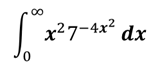3 M
1(b) Solve (D4+4)y=0
3 M
1(c) Prove that E∇ = Δ =∇E
3 M
1(d) Solve the following: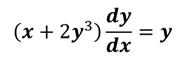3 M
1(e) Evaluate ∫∫r3drdθ over the regions between the circles r=2sinθ and r=4sinθ
4 M
1(f) Evaluate the following: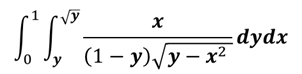4 M

2(a) Solve (x3y4+x2y3+xy2+y)dx + (x4y3-x3y2-x2y+x)dy=0
6 M
2(b) Change the order of integral and evaluate: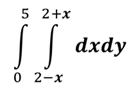6 M
2(c) Prove that: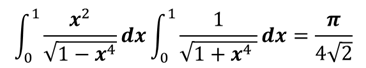8 M

3(a) Evaluate the following: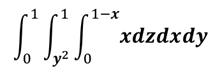6 M
3(b) Find the area of one loop of the lemniscate r2=a2cos2θ
6 M
3(c) Solve (D3+2D2+D)y = x2e3x+sin2x+2x
8 M

4(a) Show that the length of the arc of the parabola y2=4ax cut off by the line 3y=8x is alog2+15/16
6 M
4(b) Using the method of variation of parameters, solve: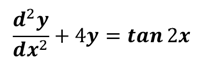6 M
4(c) Compute y(0.2) given (dy/dx)+y+xy2=0, y(0)=0, By taking h=0.1 using Runge-Kutta method of fourth order correct to 4 decimals.
8 M

5(a) Solve the following: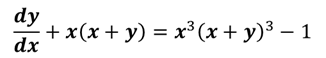6 M
5(b) Solve (dy/dx)-2y=3ex,y(0)=0 using Taylor Series method. Find approximate value of y for x=0.1 and 0.2
6 M
5(c) Evaluate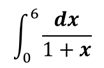using Trapezoidal rule, Simpson's 1/3rd rule and Simpson's 3/8th rule. Compare the result with exact values.
8 M

6(a) The current in a circuit containing an inductance L, resistance R and a voltage Esinωt is given by L (di/dt)+Ri=E sinωt. If i=0 at t=0, find i.
6 M
6(b) Evaluate ∫∫e(2x-3y)dxdy over the triangle bounded by x+y=1, x=1 and y=1
6 M
6(c)(1) Find the volume of solid bounded by the surfaces y2=4ax, x2=4ay and the planes z=0, z=3.
4 M
6(c)(2) Change to polar co-ordinates and evaluate: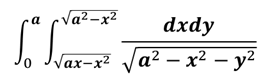4 M

More question papers from Applied Mathematics 2